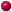# POLIP

##bip_cross_min.22.22.48.1

 Name bip_cross_min.22.22.48.1 Classification nc|bc|d2 Problem type quadratic_linear_ordering Description edge-crossing minimization in bipartite graphs modeled as a quadratic linear ordering problem Objective sense min Variables 463  (462 binary, 0 general integer, 1 continuous) Nonlinear variables 1 Constraints 6160 Nonlinear constraints 1 Linear nonzeros 18480 Nonlinear nonzeros 1358 Download bip_cross_min.22.22.48.1.pip.gz bip_cross_min.22.22.48.1.gms.gz bip_cross_min.22.22.48.1.mod.gz bip_cross_min.22.22.48.1.zpl.gz Best known solution Best known objective Best known bound Originator Christoph Buchheim, Angelika Wiegele, Lanbo Zheng Formulator Ulrike Pagacz Donator Christoph Buchheim References BuchheimWiegeleZheng2010 Links Additional information This instance is for the edge crossing minimization problem in a bipartite graph G. G has to be drawn in the plane so that the nodes of its two shores are placed on two parallel horizontal lines. The task is to minimize the number of edge crossings by permuting the order of nodes on each layer, assuming that all edges are drawn as straight lines. The problem can be modeled as a quadratic objective over linear ordering variables. The instance is from BuchheimWiegeleZheng2010.

© by maintainers  |     |  imprint